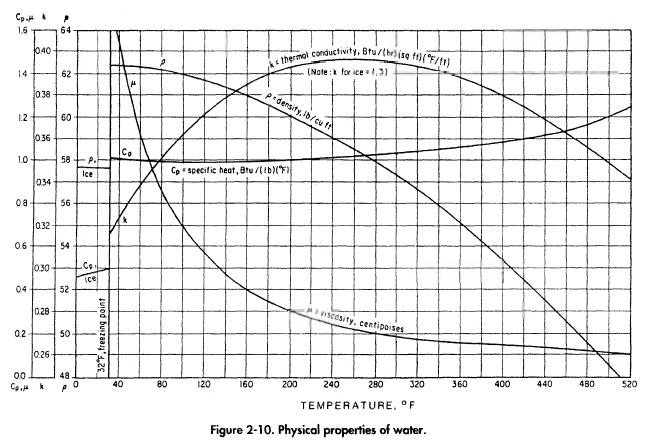﻿ Inside Film Coefficient | Oil & Gas Process Engineering

## Inside Film Coefficient

The inside film coefficient represents the resistance to heat flow caused by the change in flow regime from turbulent flow in the center of the tube to laminar flow at the tube surface. The inside film coefficient can be calculated from:

(The viscosity of a fluid in lb/hr-ft is its viscosity in centipoise times 2.41.) The bulk fluid temperature at which the fluid properties are obtained should be the average temperature between the fluid inlet and outlet temperatures. The viscosity at the tube wall should be the fluid viscosity at the arithmetic average temperature between the inside fluid bulk temperature and the tube wall temperature. The tube wall temperature may be approximated by taking the arithmetic average between the inside fluid
bulk temperature and the outside fluid bulk temperature.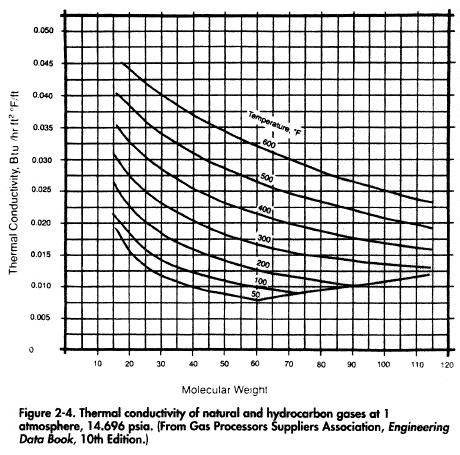The thermal conductivity of natural and hydrocarbon gases is given in Figure 2-5. The value from Figure 2-4 is multiplied by the ratio of k/k.A from Figure 2-5.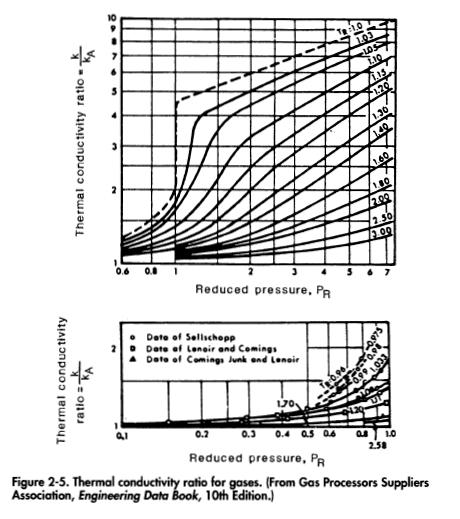The thermal conductivity of hydrocarbon liquids is given in Figure 2-6. The viscosity of natural gases and hydrocarbon liquids is discussed in Volume 1.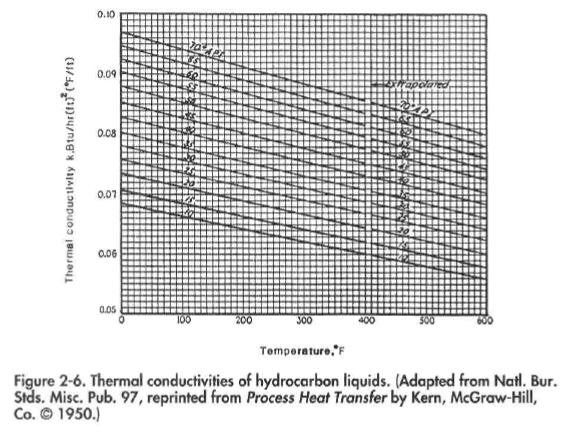The mass velocity of a fluid in pounds per hour per square foot can be calculated from

The specific heat of natural gas and hydrocarbon liquids can be calculated using procedures described later in this text (see pp. 41 and 42).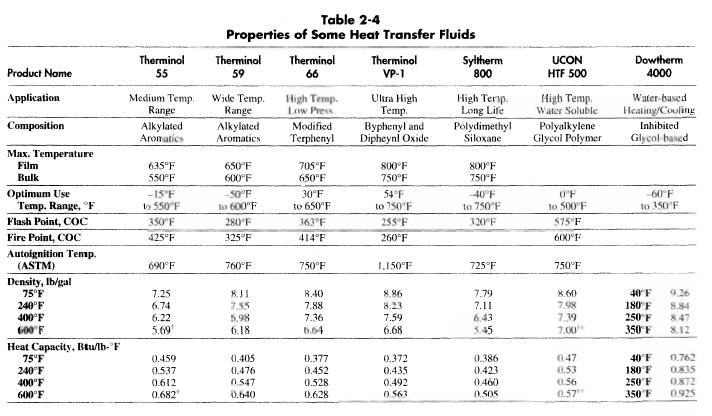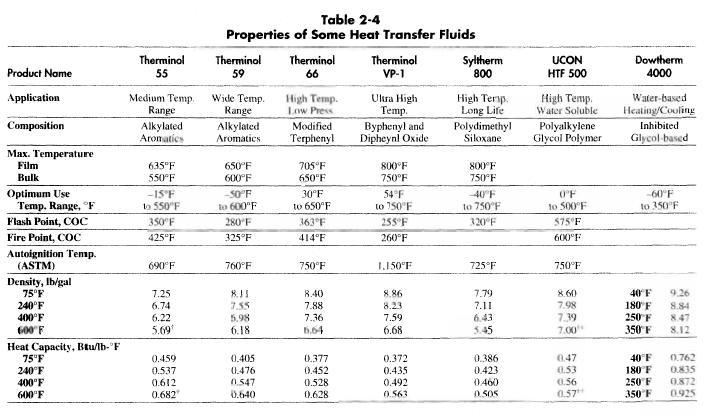The physical properties and the optimum temperature range for various heat transfer fluids are given in Table 2-4. Graphs showing more detailed physical properties and heat transfer coefficient at various conditions, such as those shown in Figures 2-7 through 2-9, can be obtained directly from manufacturers. A personal computer program for obtaining detailed physical properties of Therminol and for computing heat transfer coefficients and pressure drops in a wide variety of tube sizes and flow
conditions using Therminol as the heat transfer fluid is available from Monsanto Company.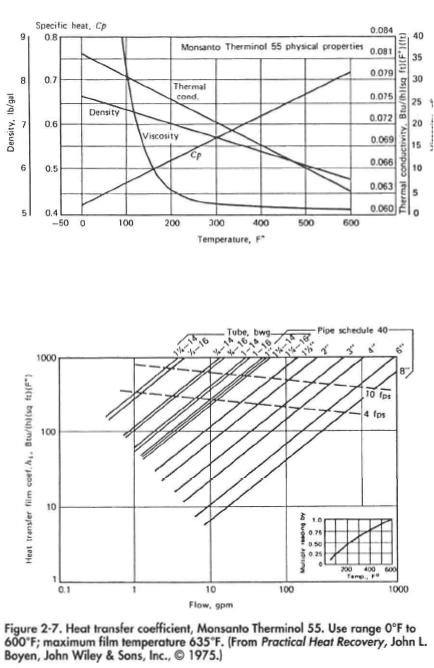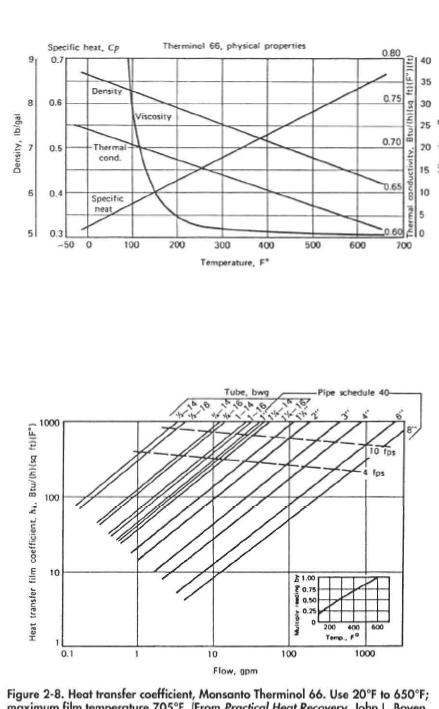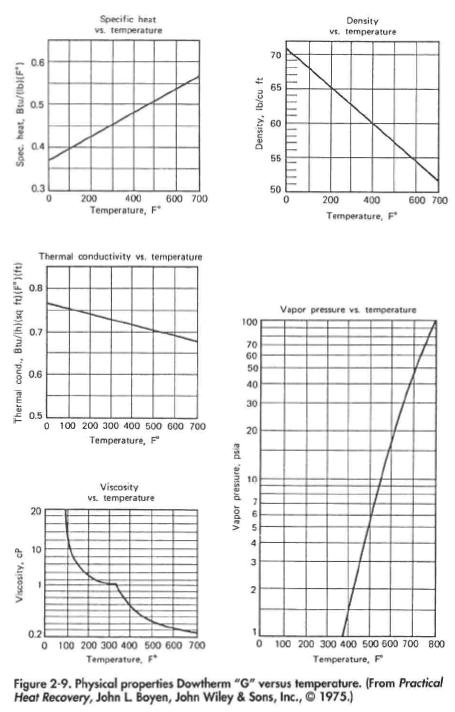Figure 2-10 gives the thermal conductivity, viscosity, and specific heat of water.